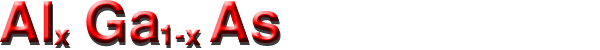## Band structure and carrier concentration

Basic Parameters
Temperature Dependences
Dependence on Hydrostatic Pressure
Energy Gap Narrowing at High Doping Levels
Band Discontinuities at AlxGa1-xAs/GaAs Heterointerface

### Basic Parameters

 Energy gap x<0.45      1.424+1.247x eV x>0.45      1.9+0.125x+0.143x2 Energy separation (EΓL) between Γ and L valleys 0.29 eV Energy separation (EΓ) between Γ and top of valence band 1.424+1.155x+0.37x2 eV Energy separation (EX) between X-valley and top of valence band 1.9+0.124x+0.144x2 eV Energy separation (EL) between L-valley and top of valence band 1.71+0.69x eV Energy spin-orbital splitting 0.34-0.04x eV Intrinsic carrier concentration x=0.1      2.1·105 cm-3 x=0.3      2.1·103 cm-3 x=0.5      2.5·102 cm-3 x=0.8      4.3·101 cm-3 Intrinsic resistivity x=0.1      4·109 Ω·cm x=0.3      1·1012 Ω·cm x=0.5      1·1014 Ω·cm x=0.8      5·1014 Ω·cm Effective conduction band density of states x<0.41      2.5·1019·(0.063+0.083x)3/2 cm-3 x>0.45      2.5·1019·(0.85-0.14x)3/2 cm-3 Effective valence band density of states 2.5·1019·(0.51+0.25x)3/2 cm-3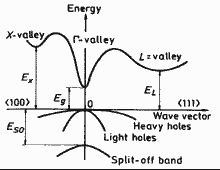Band structure AlxGa1-x for x<0.41-0.45. Important minima of the condition band and maxima of the valence band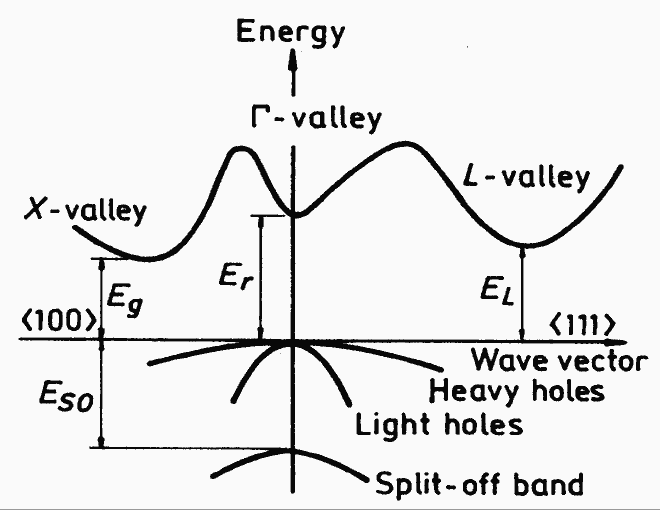Band structure AlxGa1-x for x>0.45. Important minima of the condition band and maxima of the valence band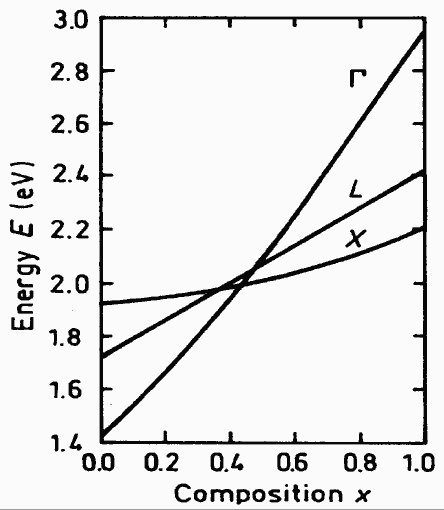Energy separation between Γ-, X-, and L- conduction band minima and top of the valence band versus composition.
Crossover points:
 xc(L-X) = 0.35 eV EL=EX = 1.95 eV xc(Γ-X) = 0.41 eV EΓ=EX = 1.97 eV xc(Γ-L) = 0.47 eV EΓ=EL = 2.04 eV
(Saxena ).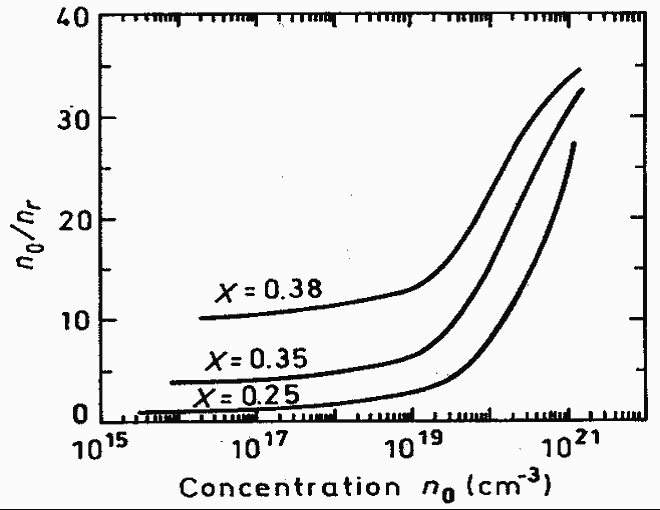Ratio of the total carrier concentration to the carrier concentration in Γ-valley as a function of equilibrium carrier concentration at 300K (Zarem et al. ).

### Temperature Dependences

To estimate the temperature dependences of energy difference between the top of the valence band and the bottom of the Γ, X, and L valleys of the conduction band EΓ, EX and EL one can use the data for GaAs (Aspnes ).

EΓ=EΓ(0)-5.41·10-4·T2/(T+204) (eV)
where EΓ(0)=1.519+1.155x+0.37x2 (eV)

EX=EX(0)-4.6·10-4·T2/(T+204) (eV)

where EX(0)=1.981+0.124x+0.144x2 (eV)

EL=EL(0)-6.05·10-4·T2/(T+204) (eV)

where EL(0)=1.815+0.0.69x (eV)

Temperature dependence of the energy difference between the top of the valence band and the bottom of the L-valley of the conduction band

EL=1.815-6.05·10-4·T2/(T+204) (eV)

Temperature dependence of the energy difference between the top of the valence band and the bottom of the X-valley of the conduction band

EL=1.981-4.60·10-4·T2/(T+204) (eV)

#### Effective density of states in the conduction band Nc

X<0.41    Nc=4.82·1015·(mΓ/mo)3/2·T3/2 = 4.82·1015·T3/2·(0.063+0.083x)3/2 (cm-3)

X>0.41    Nc=4.82·1015·(mcd/mo)3/2·T3/2 = 4.82·1015·T3/2·(0.85-0.14x)3/2 (cm-3)

where mcd is effective mass of the density of states;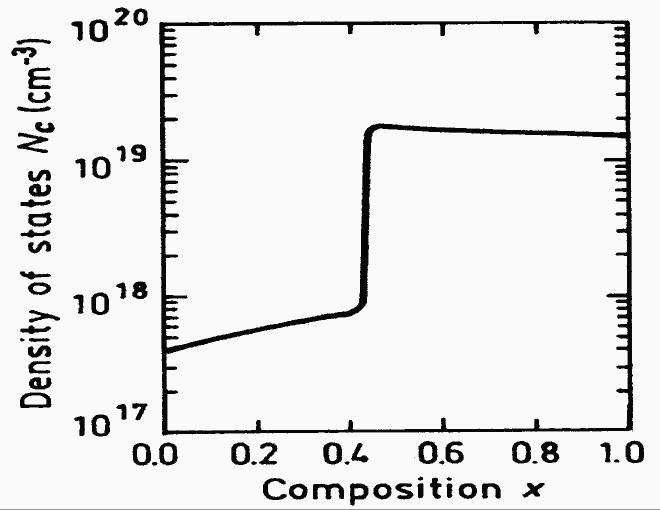Effective density of states in the conduction band versus x.(Calculated)

#### Effective density of states in the valence band Nv

Nv = 4.82·1015·T3/2·(0.51+0.25x)3/2 (cm-3) X>0.41    Nc=4.82·1015·(mcd/mo)3/2·T3/2 = 4.82·1015·T3/2·(0.85-0.14x)3/2 (cm-3)Effective density of states in the conduction band versus x.(Calculated)

#### Intrinsic Carrier Concentration

ni = (Nc·Nv)1/2exp[-Eg/(2kbT)]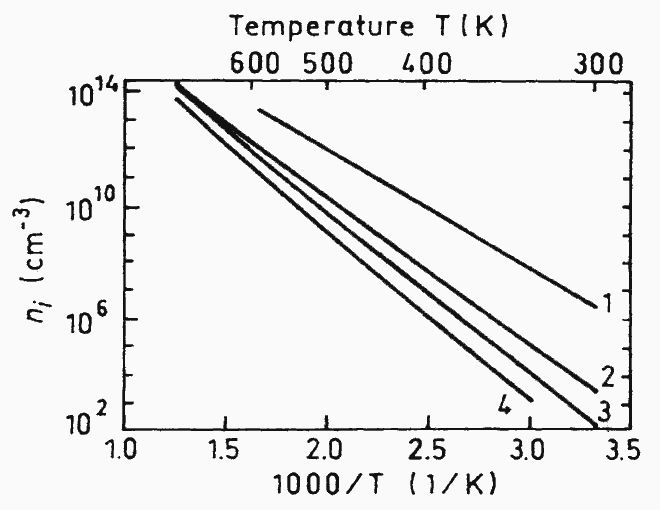The temperature dependences of the intrinsic carrier concentration. 1. x=0 2. x=0.3 3. x=0.6 4. x=1

### Dependences on Hydrostatic Pressure

EΓ = (11.5 - 1.3 x)·10-3·P (eV)
EX = -0.8·10-3·P (eV)
EL = 2.8·10-3·P (eV)
where P is pressure in kbar. (Adachi )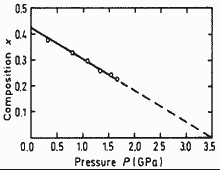Pressure dependence of the Γ-X crossover. 300 K (Saxena )

### Energy Gap Narrowing at High Doping Levels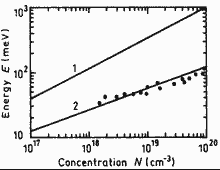Energy gap narrowing versus donor(curve 1) and acceptor (curve 2) doping density for GaAs (x=0). Experimental points for p-GaAs are taken from four different papers (Jain and Roulston )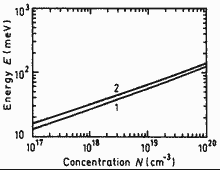Energy gap narrowing versus donor (curve 1) and acceptor (curve 2)doping density for AlAs (x=1). The curvesare calculated according (Jain et al. )

### Band Discontinuities at AlxGa1-xAs/GaAs Heterointerface

#### Valence band discontinuity:

ΔEv = - 0.46x (eV)

#### Conduction band discontinuity:

x<0.41       ΔEc = 0.79x (eV)
x>0.41       ΔEc = 0.475-0.335x+0.143x2 (eV)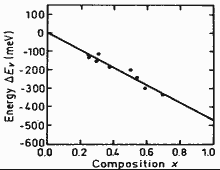Energy gap narrowing versus donor(curve 1) and acceptor (curve 2) doping density for GaAs (x=0). Experimental points for p-GaAs are taken from four different papers (Jain and Roulston )